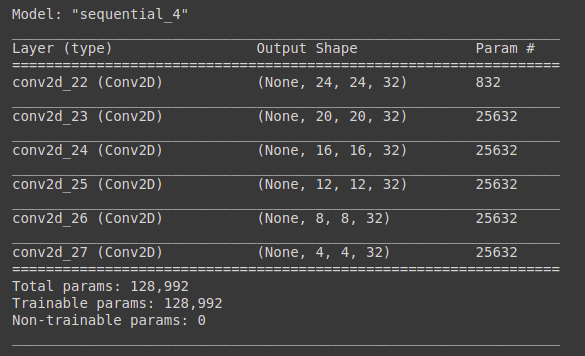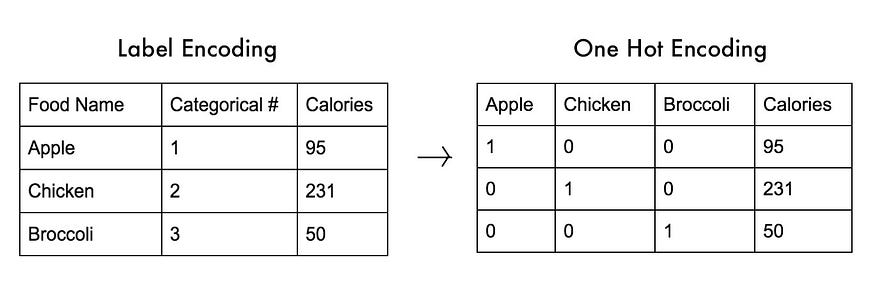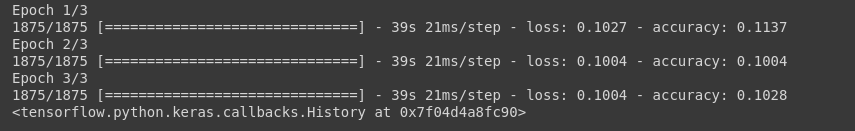# Beginners Guide to Debugging TensorFlow Models

If you are new to working with a deep learning framework, such as TensorFlow, there are a variety of typical errors beginners face when building and training models. Here, we explore and solve some of the most common errors to help you develop a better intuition for debugging in TensorFlow.Photo by Dmitriy Demidov on Unsplash.

TensorFlow is one of the most famous deep learning models, and it is easy to learn. This article will discuss the most common errors a beginner can face while learning TensorFlow, the reasons, and how to solve these errors. We will discuss the solutions and also what experts from StackOverflow say about them.

### Example 1: Wrong Input Shape for CNN layer

Suppose you are making a Convolutional Neural Network, now if you are aware of the theory of CNN, you must know that a CNN (2D) takes in a complete image as its input shape. And a complete image has 3 color channels that are red, green, black. So the shape of a normal image would be (height, width, color channels). But if you pass in a grayscale image, it is normally (height, width), and the color channel is excluded as shown in the code.

```model = Sequential([
Conv2D(32, 5, input_shape=(28,28), activation=’relu’),
Flatten(),
Dense(10, activation=’softmax’)
])

```

Now, if you train this model, you would get an error.

```ValueError: Input 0 of layer conv2d_1 is incompatible with the layer: : expected min_ndim=4, found ndim=3. Full shape received: (None, 28, 28)

```

This is because we are passing the input shape of (28,28) and 1 extra dimension added by TensorFlow for Batch size, so the error message says that it found ndim=3, but the CNN has expected min_ndim=4, 3 for the image size and 1 for the batch size.

So you can solve this error by changing the input shape in the first CNN layer and reshaping your inputs before passing it to CNN.

```X_train=x_train.reshape(number_of_rows,28,28,1)

```

This will change your input from (number_of_rows, height, width) to (number_of_rows, height, width, color_channel) where color_channel is equal to 1, showing that it is a grayscale image. Now your CNN is ready to work. You can check this StackOverflow question for more details.

### Example 2: Negative Dimension Size

This is one of the common errors that new practitioners deal with when playing with CNNs or other models that change input shape after each layer. Now the output shape of CNN depends on several factors such as the number of filters, kernel size, padding type, and stride size. Let’s say you have a model:

```model = Sequential([
Conv2D(32, 5, input_shape=(28,28,1), activation='relu'),
Conv2D(32, 5, activation='relu'),
Conv2D(32, 5, activation='relu'),
Conv2D(32, 5, activation='relu'),
Conv2D(32, 5, activation='relu'),
Conv2D(32, 5, activation='relu'),
])

model.summary()

```You can see that the input size is getting smaller and smaller, and if you add any more CNN layers, it will reduce to negative and hence raise a negative dimension error. So you need to understand how tuning different CNN parameters will affect your output shape. The error message after adding another CNN layer would be a long traceback message, but you need to find the right part of it, that is:

```ValueError: Negative dimension size caused by subtracting 5 from 4 for ‘{{node conv2d_16/Conv2D}} = Conv2D[T=DT_FLOAT, data_format=”NHWC”, dilations=[1, 1, 1, 1], explicit_paddings=[], padding=”VALID”, strides=[1, 1, 1, 1], use_cudnn_on_gpu=true](Placeholder, conv2d_16/Conv2D/ReadVariableOp)’ with input shapes: [?,4,4,32], [5,5,32,32].

```

There are different solutions to it, such as changing the padding or stride size, changing the number of layers, and tuning the other hyperparameters while keeping the output shape of that layer in your mind. You can have a look at a detailed discussion on it on StackOverflow here and here, where several good solutions with reasons are offered.

### Example # 3: Wrong Output Shape

This is also a common error that beginners face of having the wrong number of nodes in the last layer. In the last layer of any neural network, you need to have the number of nodes equal to the number of classes you have or the number of outputs you want. For example, in a regression task, you normally have 1 node in the output layer because you need a single continuous value as output. In the classification task, you have n number of nodes in the output layers, which is equal to the total number of unique classes.

Let’s say you have 10 unique classes in your example, and you specify 9 in your output layer as follows:

```model = Sequential([
Conv2D(32, 5, input_shape=(28,28,1), activation=’relu’),
MaxPool2D((2,2)),
Conv2D(32,3, activation=’relu’),
Flatten(),
Dense(9, activation=’softmax’)
])

```

Now when you train your model after compiling it, it will raise an error message.

```model.compile(‘adam’,’categorical_crossentropy’, [‘acc’])

model.fit(X_train, y_train, epochs=3)

```

Error Message:

```ValueError: Shapes (32, 10) and (32, 9) are incompatible

```

And you can see that as the error message says, shapes are not compatible. You can have a look at this thread in StackOverflow for more details regarding this error.

### Example # 4: Unknown Loss function

This error, as the name shows, generates when you have used a loss function that does not exist in Tensorflow.

Let’s say you compile a model

```model.compile('adam','sparse_categorical_crossentropy', ['acc'])
```

Now you have used the sparse_categorical_crossentropy loss function here, which does not exist due to wrong spelling, and a lot of beginners make similar spelling mistakes. Now the tricky part is that you will not get the error message on the compilation. In fact, you will get the error message when you fit the model.

```model.fit(X_train, y_train, epochs=1)

```

Now you will receive the long error message, which you can trace and find useful information from it.

```ValueError Traceback (most recent call last)
— -> 11 model.fit(X_train, y_train, epochs=1)
#Long Error traceback
ValueError: Unknown loss function: sparse_categoricalcrossentropy

```

### Example #5 Shape not compatible with appropriate function

This is a very common error message, and it appears when you are using a function or a layer or anything similar, and it expects a specific type of shape, but the shape you are passing is different from the required shape. All of these will generate a related or similar error message that is ValueError: Shape mismatch:

Let’s see an example.

Suppose your output labels are in one-hot-matrix format.Credits: Medium.

Now, these output labels require a special loss function for simple classification tasks that is categorical_crossentropy, and if you pass in sparse_categorcial_crossentropy that is used for Label Encoded Output labels, this will generate a shape-mismatch error.

```model = Sequential([
Conv2D(32, 5, input_shape=(28,28,1), activation=’relu’),
MaxPool2D((2,2)),
Conv2D(32,3, activation=’relu’),
Flatten(),
Dense(10, activation=’softmax’)
])
model.fit(X_train, y_train, epochs=1)

```

Since our output y_train is in the one-hot matrix format, it will generate an error.

```ValueError: Shape mismatch: The shape of labels (received (320,)) should equal the shape of logits except for the last dimension (received (32, 10)).

```

This error means that the sparse categorical cross-entropy loss function expects a single vector, and we are passing a matrix.

### Example # 6 Wrong Loss function

This is not an error, but rather a mistake where your model’s performance would not be improving and giving very bad results. While there can be many different reasons for it, a common reason behind it is using the wrong loss function. For example, in classification tasks, you are supposed to use the cross-entropy or related loss function, and if you are using a loss function that is not suitable for classification tasks, then your model will not improve.

```model = Sequential([
Conv2D(32, 5, input_shape=(28,28,1), activation='relu'),
MaxPool2D((2,2)),
Conv2D(32,3, activation='relu'),
Flatten(),
Dense(10)
])
model.compile('rmsprop','mae', ['accuracy'])
model.fit(X_train, y_train, epochs=3)

```

Here we are using the mean absolute error loss function instead of the cross-entropy loss function, and as a result of this, our model is not performing.You can see the accuracy stuck at 10% and loss is also not improving. If you are stuck at a similar stage where your model is not improving, I suggest you take a look at every single step back where you have specified things and think upon what can be the possible problem due to which my model is not training correctly, because you are not going to see any error message in this case. Also, you can ask questions at any community, such as r/learnmachinelearning on Reddit or StackOverflow.

Related: